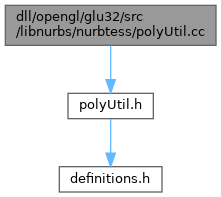ReactOS  0.4.15-dev-5606-gf34e425
polyUtil.cc File Reference
`#include "polyUtil.h"`
Include dependency graph for polyUtil.cc:Go to the source code of this file.

## Functions

Real area (Real A, Real B, Real C)

Int pointLeftLine (Real A, Real B, Real P)

Int pointLeft2Lines (Real A, Real B, Real C, Real P)

## ◆ area()

 Real area ( Real A, Real B, Real C )

Definition at line 43 of file polyUtil.cc.

44 {
45  Real Bx, By, Cx, Cy;
46  Bx = B - A;
47  By = B - A;
48  Cx = C - A;
49  Cy = C - A;
50  return Bx*Cy - Cx*By;
51
52 /* return (B-A)*(C-A) - (C-A)*(B-A);*/
53 }
Definition: ehthrow.cxx:53
Definition: terminate.cpp:23
Definition: ehthrow.cxx:92
float Real
Definition: definitions.h:36

Referenced by pointLeft2Lines(), and pointLeftLine().

## ◆ pointLeft2Lines()

 Int pointLeft2Lines ( Real A, Real B, Real C, Real P )

Definition at line 78 of file polyUtil.cc.

79 {
80  Int C_left_AB = (area(A, B, C)>0);
81  Int P_left_AB = (area(A, B, P)>0);
82  Int P_left_BC = (area(B, C, P)>0);
83
84  if(C_left_AB)
85  {
86  return (P_left_AB && P_left_BC);
87  }
88  else
89  return (P_left_AB || P_left_BC);
90 }
Definition: ehthrow.cxx:53
Definition: terminate.cpp:23
Definition: ehthrow.cxx:92
int Int
Definition: definitions.h:37
#define P(row, col)
Real area(Real A, Real B, Real C)
Definition: polyUtil.cc:43

Referenced by MC_partitionY(), and partitionY().

## ◆ pointLeftLine()

 Int pointLeftLine ( Real A, Real B, Real P )

Definition at line 64 of file polyUtil.cc.

65 {
66  if(area(A, B, P) >0) return 1;
67  else return 0;
68 }
Definition: ehthrow.cxx:53
Definition: ehthrow.cxx:92
#define P(row, col)
Real area(Real A, Real B, Real C)
Definition: polyUtil.cc:43